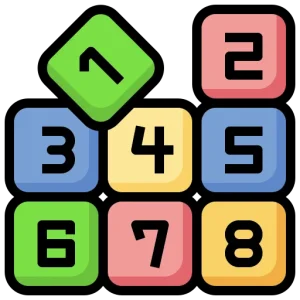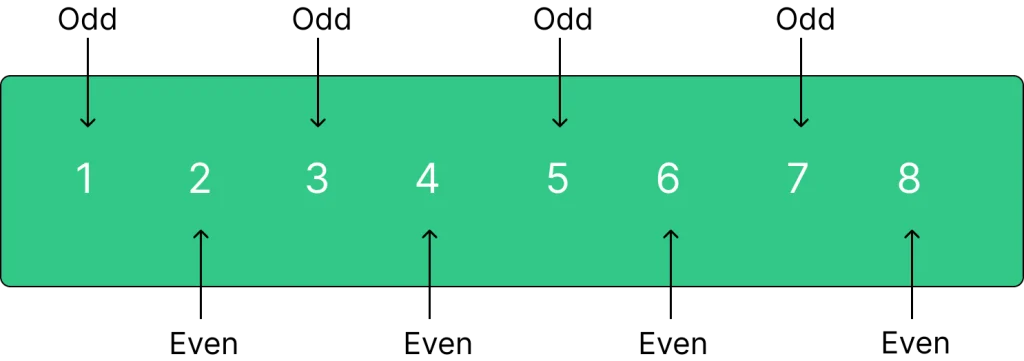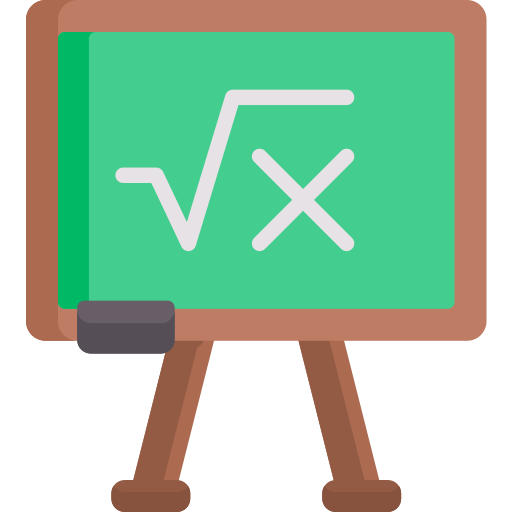# Python program to check if a number is Odd or Even## Introduction

In Python, you can easily determine whether a number is odd or even using a simple conditional statement. In this tutorial, we will show you how to create a Python program that checks the parity of a given number.

Understanding how to check if a number is odd or even is not only a fundamental concept but also a building block for more complex programs. By the end of this tutorial, you’ll have a clear grasp of the necessary Python syntax and logic required to tackle this problem. So, let’s dive in and learn.

## Concept of Even Number and Odd Number

###### Even Number

An even number is any integer that is exactly divisible by 2, meaning that when you divide it by 2, there is no remainder.

Examples of even numbers include 2, 4, 6, 8, 10, and so on.

###### Odd Number

An odd number is any integer that is not exactly divisible by 2, meaning that when you divide it by 2, there is a remainder.

Examples of odd numbers include 1, 3, 5, 7, 9, and so on.

## Example :Example 1:

Input: num = 12
Output: Even

Example 2:

Input: n = 9
Output: Odd

## Python program to check if a number is odd or even

Here’s a code snippet :

```# Input a number from the user
num = int(input("Enter a number: "))

# Check if the number is even or odd
if num % 2 == 0:
print(str(num) + " is even.")
else:
print(str(num) + " is odd.")
```

### Output :

```Enter a number: 10
10 is even.
```

### Explanation :

This Python program checks whether a user-inputted number is odd or even. It takes a number as input, calculates the remainder when divided by 2, and based on the remainder, it determines whether the number is odd or even. The result is then displayed as output.

## Python program to check if a number is odd or even using Recursion

Here’s a code snippet :

```def is_even(num):
if num == 0:
return True
elif num == 1:
return False
else:
return is_even(num - 2)

# Input a number from the user
num = int(input("Enter a number: "))

# Check if the number is even or odd using recursion
if is_even(num):
print(str(num) + " is even.")
else:
print(str(num) + " is odd.")
```

### Output :

```Enter a number: 10
10 is even.
```

### Explanation :

This Python program uses recursion to check if a user-inputted number is odd or even. It defines a recursive function that subtracts 2 from the number until it reaches 0 (indicating an even number) or 1 (indicating an odd number). The program then displays the result, providing information to the user. It offers an alternative approach to determine the parity of a number, showcasing the use of recursion in Python.

## Python program to check if a number is odd or even using function

Here’s a code snippet :

```# Define a function to check if a number is odd or even
def check_odd_even(num):
if num % 2 == 0:
return "even"
else:
return "odd"

# Input a number from the user
num = int(input("Enter a number: "))

# Call the function to check if the number is odd or even
result = check_odd_even(num)

# Display the result
print(str(num) + " is " + result + ".")
```

### Output :

```Enter a number: 18
18 is even.
```

### Explanation :

This Python program uses a function to determine whether a user-inputted number is odd or even. The check_odd_even function checks for evenness using the modulo operator and returns a string indicating “even” or “odd.” The user inputs a number, and the program calls the function to check its parity. The result is displayed using string concatenation. This provides a clear explanation of whether the number is even or odd.

## Complexity analysis for checking Odd or Even

Time Complexity:

• The time complexity of this program is constant, O(1), because the number of operations does not depend on the size of the input.

Space Complexity:

• The space complexity is also constant, O(1), as the program uses a fixed amount of memory regardless of the input.
##### To wrap it up:

In this Python program, we have created a simple yet powerful tool to distinguish between even and odd numbers. Understanding the concept of even and odd numbers is fundamental in mathematics and has practical applications across various fields, including programming, data analysis, finance, and more.

### Related Banners

Get PrepInsta Prime & get Access to all 200+ courses offered by PrepInsta in One SubscriptionQuestion 1.

What is the purpose of checking if a number is odd or even in programming?

Checking whether a number is odd or even is a fundamental concept used in various programming scenarios. It is commonly used for controlling program flow, categorizing data, or implementing specific logic based on the parity of numbers.Question 2.

Can I use this program to check numbers other than integers?

This program is designed to check the parity of integers. If you input non-integer values, the program may produce unexpected results or errors.Question 3.

Can I modify this program to check for other properties of numbers?

Yes, you can adapt this program to check for additional properties of numbers, such as prime or composite numbers. By modifying the conditional statements, you can create programs to suit your specific needs.

## Get over 200+ course One Subscription

Courses like AI/ML, Cloud Computing, Ethical Hacking, C, C++, Java, Python, DSA (All Languages), Competitive Coding (All Languages), TCS, Infosys, Wipro, Amazon, DBMS, SQL and others